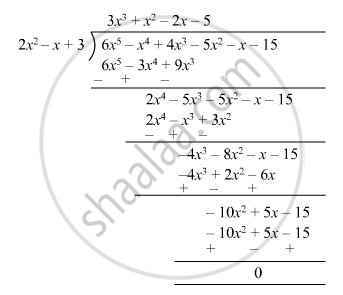Advertisement Remove all ads

# Using Division of Polynomials, State Whether 2x2 − X + 3 is a Factor of 6x5 − X4 + 4x3 − 5x2 − X − 15 - Mathematics

Sum

Using division of polynomials, state whether

2x2 − x + 3 is a factor of 6x5 − x4 + 4x3 − 5x2 − x − 15

Advertisement Remove all ads

#### SolutionRemainder is zero ; therefore,

$2 x^2 - x + 3$  is a factor of$6 x^5 - x^4 + 4 x^3 - 5 x^2 - x - 15$
Is there an error in this question or solution?
Advertisement Remove all ads

#### APPEARS IN

RD Sharma Class 8 Maths
Chapter 8 Division of Algebraic Expressions
Exercise 8.4 | Q 23.6 | Page 12
Advertisement Remove all ads

#### Video TutorialsVIEW ALL 

Advertisement Remove all ads
Share
Notifications

View all notifications

Forgot password?
Course